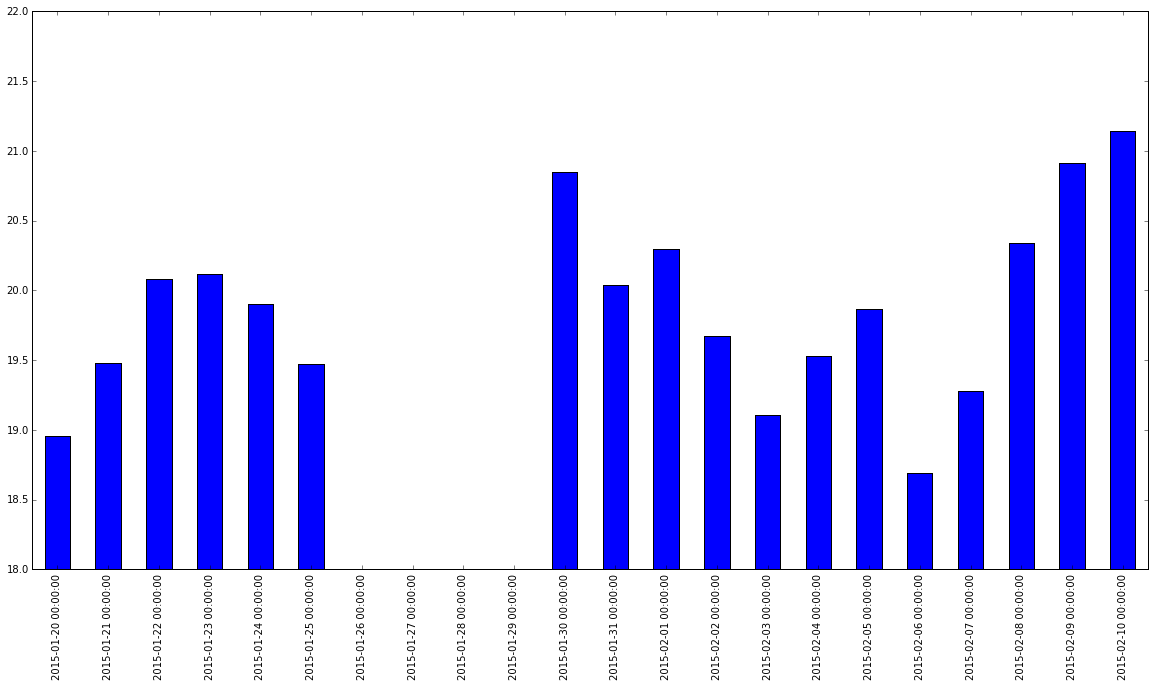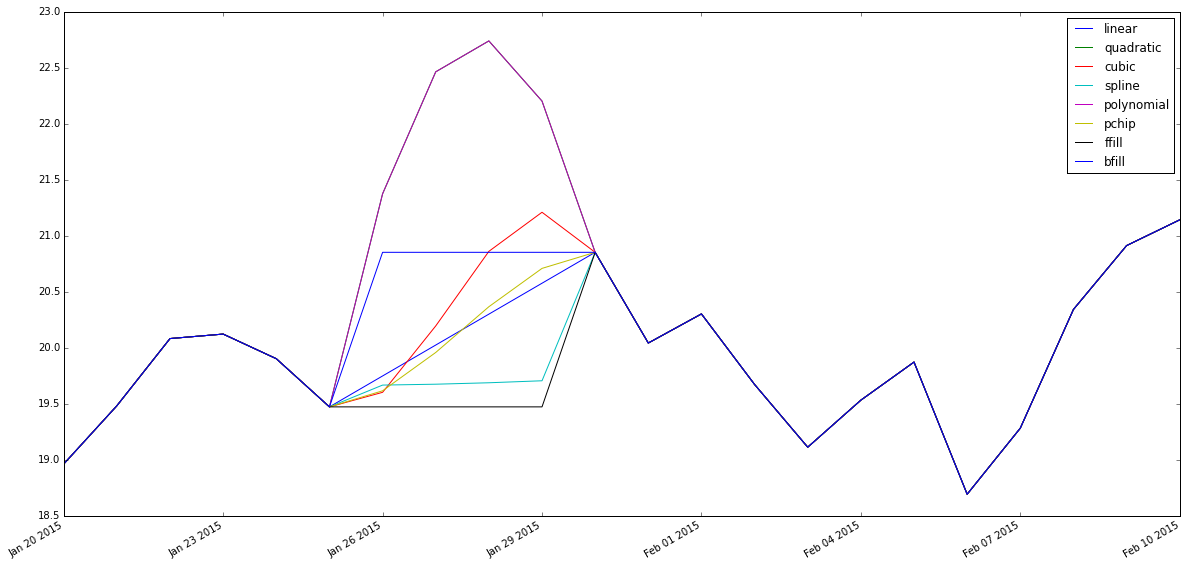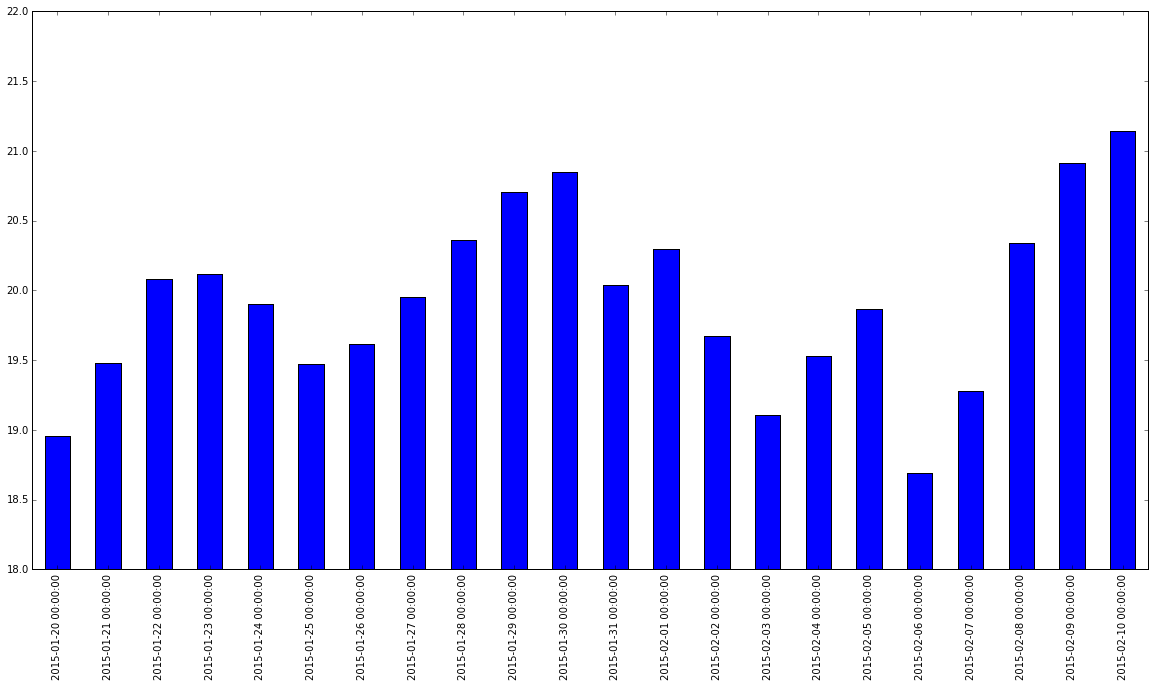# Interpolating data with Python

SensorData

So as usual for this time of year I find myself on vacation with very little to do. So I try and find personal projects that interest me. This is usually a mixture of electronics and mucking around with software in a way that I don't usally find the time for normally. One of projects is my sensor network.

I have a number of Raspberry Pi's around my house and garden that take measurements of temperature, humidity, pressure and light. They hold the data locally and then periodically upload them to a central server (another Raspberry Pi) where they are aggregated. However for any number of reasons (usally a power failure) the raspberrypi's occasionally restart and are unable to join the network. This means that some of their data is lost. I've improved their resiliance to failure and so it's a less common occurance but it's still possible for it to happen. When this means I'm left with some ugly gaps in an otherwise perfect data set. It's not a big deal but it's pretty easy to fix. Before I begin, I acknolwedge that I'm effectively "making up" data to make graphs "prettier".

In the following code notebook I'll be using Python and Pandas to tidy up the gaps.

To start with I need to load the libraries to process the data. The important ones are included at the start of the imports. The rest from "SensorDatabaseUtilities" aren't really relevant since they are just helper classes to get data from my repository

In :
import matplotlib.pyplot as plt
from matplotlib import style
import pandas as pd
import matplotlib
import json
from pandas.io.json import json_normalize
# The following imports are from my own Sensor Library modules and aren't really relevant
from SensorDatabaseUtilities import AggregateItem
from SensorDatabaseUtilities import SensorDatabaseUtilities
# Make sure the charts appear in this notebook and are readable
%matplotlib inline
matplotlib.rcParams['figure.figsize'] = (20.0, 10.0)


The following function is used to convert a list of JSON documents (sensor readings) into a Pandas DataFrame. It then finds the minimum and maximum dates and creates a range for that period. It uses this period to find any missing dates. The heavy lifting of the function uses the reindex() function to insert new entries whilst at the same time interpolating any missing values in the dataframe. It then returns just the newly generated rows

In :
def fillin_missing_data(sensor_name, results_list, algorithm='linear', order=2):
# Turn list of json documents into a json document
results = {"results": results_list}
# Convert JSON into Panda Dataframe/Table
df = json_normalize(results['results'])
# Convert Date String to actual DateTime object
df['Date'] = pd.to_datetime(df['Date'])
# Find the max and min of the Data Range and generate a complete range of Dates
full_range = pd.date_range(df['Date'].min(), df['Date'].max())
# Find the dates that aren't in the complete range
missing_dates = full_range[~full_range.isin(df['Date'])]
# Set the Date to be the index
df.set_index(['Date'], inplace=True)
# Reindex the data filling in the missing date and interpolating missing values
if algorithm in ['spline', 'polynomial'] :
df = df.sort_index().reindex(full_range).interpolate(method=algorithm, order=order)
elif algorithm in ['ffill', 'bfill']:
df = df.sort_index().reindex(full_range, method=algorithm)
else:
df = df.sort_index().reindex(full_range).interpolate(method=algorithm)
# Find the dates in original data set that have been added
new_dates = df[df.index.isin(missing_dates)]
# Create new aggregate records and insert them into the database
# new_dates.apply(gen_json,axis=1, args=[sensor_name])
return new_dates


This function simply takes an array of JSON documents and converts them into a DataFrame using the Pandas json_normalize function. It provides us with the dataset that contains missing data i.e. an incomplete data set.

In :
def json_to_dataframe(results_list):
# Turn list of json documents into a json dodument
results = {"results": results_list}
# Convert JSON into Panda Dataframe/Table
df = json_normalize(results['results'])
return df


The first step is to pull the data from the database. I'm using some helper functions to do this for me. I've also selected a date range where I know I have a problem.

In :
utils = SensorDatabaseUtilities('raspberrypi', 'localhost')
data = utils.getRangeData('20-jan-2015', '10-feb-2015')
# The following isn't need in the code but is included just to show the structure of the JSON Record
json.dumps(data)

Out:
'{"Date": "2015-01-20 00:00:00", "AverageHumidity": 35.6, "AverageTemperature": 18.96, "AveragePressure": 99838.78, "AverageLight": 119.38}'

Next simply convert the list of JSON records into a Pandas DataFrame and set it's index to the "Date" Column. NOTE : Only the first 5 records are shown

In :
incomplete_data = json_to_dataframe(data)
# Find the range of the data and build a series with all dates for that range
full_range = pd.date_range(incomplete_data['Date'].min(), incomplete_data['Date'].max())
incomplete_data['Date'] = pd.to_datetime(incomplete_data['Date'])
incomplete_data.set_index(['Date'], inplace=True)
# Show the structure of the data set when converted into a DataFrame

Out:
AverageHumidity AverageLight AveragePressure AverageTemperature
Date
2015-01-20 35.60 119.38 99838.78 18.96
2015-01-21 38.77 63.65 99617.15 19.48
2015-01-22 37.45 143.00 100909.08 20.08
2015-01-23 35.52 119.87 101306.30 20.12
2015-01-24 39.72 92.43 101528.54 19.90

The following step isn't needed but simply shows the problem we have. In this instance we are missing the days for Janurary 26th 2015 to Janurary 30th 2015

In :
#incomplete_data.set_index(['Date'], inplace=True)
problem_data = incomplete_data.sort_index().reindex(full_range)
axis = problem_data['AverageTemperature'].plot(kind='bar')
axis.set_ylim(18,22)
plt.show()Pandas offers you a number of approaches for interpolating the missing data in a series. They range from the simple method of backfilling or forward filling values to the more powerful approaches of methods such as "linear", "quadratic" and "cubic" all the way through to the more sophisticated approaches of "pchip", "spline" and "polynomial". Each approach has its benefits and disadvantages. Rather than talk through each it's much simpler to show you the effect of each interpolation on the data. I've used a line graph rather than a bar graph to allow me to show all of the approaches on a single graph.

In :
interpolation_algorithms = ['linear', 'quadratic', 'cubic', 'spline', 'polynomial', 'pchip', 'ffill', 'bfill']

fig, ax = plt.subplots()
for ia in interpolation_algorithms:
new_df = pd.concat([incomplete_data, fillin_missing_data('raspberrypi', data, ia)])
ax = new_df['AverageTemperature'].plot()

handles, not_needed = ax.get_legend_handles_labels()
ax.legend(handles, interpolation_algorithms, loc='best')

plt.show()Looking at the graph it appears that either pchip (Piecewise Cubic Hermite Interpolating Polynomial) or Cubic interpolation is going to provide the best approximation for the missing values in my data set. This is largely subjective because these are "made up values" but I believe either of these approaches provide values that are closest to what the data could have been.

The next step is to apply one to the incomplete data set and store it back in the database

In :
complete_data = pd.concat([incomplete_data, fillin_missing_data('raspberrypi', data, 'pchip')])

axis = complete_data.sort_index()['AverageTemperature'].plot(kind='bar')
axis.set_ylim(18,22)
plt.show()And thats it. I've made the code much more verbose that it needed to be purely to demonstrate the point. Pandas makes it very simple to patch up a data set.